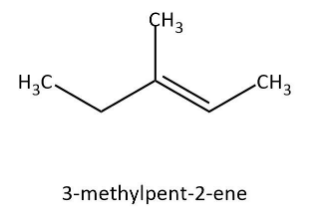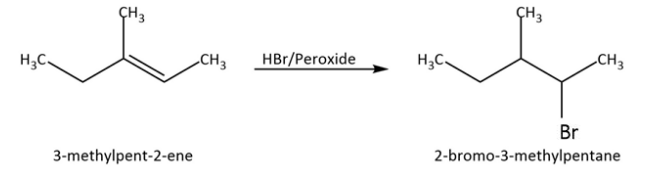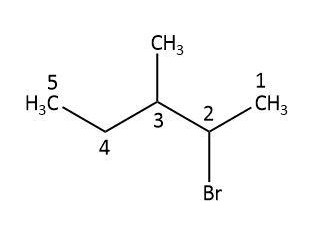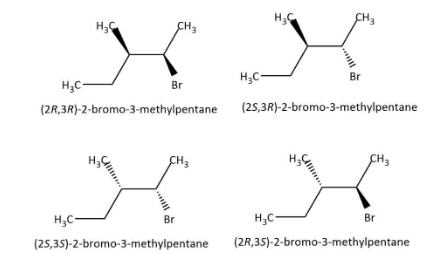# $3 - {\rm{methylpent}} - 2 - {\rm{ene}}$ on reaction with ${\rm{HBr}}$ in presence of peroxide forms an addition product. The number of possible stereoisomers for the products:(A) Zero(B) Two(C) Four(D) SixVerified
177.6k+ views
Hint:We know that in case peroxide is present, addition of ${\rm{HBr}}$ follows anti-Markovnikov rule and number of possible stereoisomers can be deduced from the number of chiral centres.

Complete step-by-step answer:Let’s start by having a look at our given alkene that is $3 - {\rm{methylpent}} - 2 - {\rm{ene}}$. We can draw the structure for this alkene as follows:As we can see that it is an unsymmetrical alkene, so we have to be careful with the addition of ${\rm{HBr}}$ to this. Usually we would follow Markovnikov rule but now we are doing addition in the presence of peroxide. So, we have to follow the anti-Markovnikov rule and we will see that less substituted carbon will get priority. Let’s write the addition reaction as follows:Now that we have our addition product, let’s find out how many chiral centres are there in this molecule by identifying carbon atoms that are bonded to four different substituents.In the addition product, we can see that the first carbon has three hydrogen atoms, second and third carbons have four different substituents, fourth has two hydrogen atoms and fifth has three hydrogen atoms. So, we can say that we have two chiral centres in our addition product.
Now we can find out the number of possible stereoisomers as ${2^2} = 4$, to be four. We can draw the structures of the four stereoisomers as follows:Hence, the correct option is C.

Note: We can understand the formation of the product according to the anti-Markovnikov rule as participation of peroxide in the free radical mechanism and giving rise to peroxide effect.